# Birefringence

Birefringence is the optical property of a material having a refractive index that depends on the polarization and propagation direction of light.

These optically anisotropic materials are said to be birefringent (or birefractive). The birefringence is often quantified as the maximum difference between refractive indices exhibited by the material.

Crystals with asymmetric crystal structures are often birefringent, as well as plastics under mechanical stress.

Birefringence is responsible for the phenomenon of double refraction whereby a ray of light, when incident upon a birefringent material, is split by polarization into two rays taking slightly different paths. This effect was first described by the Danish scientist Rasmus Bartholin in 1669, who observed it in calcite, a crystal having one of the strongest birefringences.

However it was not until the 19th century that Augustin-Jean Fresnel correctly described the phenomenon in terms of polarization, understanding light as a wave with field components in transverse polarizations (perpendicular to the direction of the wave vector).

## Explanation

The simplest (and most common) type of birefringence is that of materials with uniaxial anisotropy. That is, the structure of the material is such that it has an axis of symmetry with all perpendicular directions optically equivalent. This axis is known as the optic axis of the material, and components of light with linear polarizations parallel and perpendicular to it have unequal indices of refraction, denoted ne and no, respectively, where the subscripts stand for extraordinary and ordinary. The names reflect the fact that, if unpolarized light enters the material at some angle of incidence, the component of the incident radiation whose polarization is perpendicular to the optic axis will be refracted according to the standard law of refraction for a material of refractive index no, while the other polarization component, the so-called extraordinary ray will refract at a different angle determined by the angle of incidence, the orientation of the optic axis, and the birefringence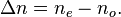$\Delta n=n_e-n_o.\,$

What’s more, the extraordinary ray is an inhomogeneous wave whose power flow (given by the Poynting vector) is not exactly parallel to the wave vector. This causes a shift in that beam, even when launched at normal incidence, that is popularly observed using a crystal of calcite as photographed above. Rotating the calcite crystal will cause one of the two images, that of the extraordinary ray, to rotate slightly around that of the ordinary ray which remains fixed.

When the light propagates either along or orthogonal to the optic axis, such a lateral shift does not occur. In the first case, both polarizations see the same effective refractive index, so there is no extraordinary ray. In the second case the extraordinary ray propagates at a different phase velocity (corresponding to ne) but is not an inhomogeneous wave.

A crystal with its optic axis in this orientation, parallel to the optical surface, may be used to create a waveplate, in which there is no distortion of the image but an intentional modification of the state of polarization of the incident wave. For instance, a quarter-wave plate is commonly used to create circular polarization from a linearly polarized source.

The more general case of biaxially anisotropic materials, also known as trirefringent[citation needed] materials, is substantially more complex. Then there are three refractive indices corresponding to three principal axes of the crystal. Generally both polarizations are extraordinary rays with different effective refractive indices which can be determined using the index ellipsoid for a given polarization vector.

## Sources of optical birefringence

While birefringence is usually obtained using an anisotropic crystal, it can result from an optically isotropic material in a few ways:

• Stress birefringence results when isotropic materials are stressed or deformed such that the isotropy is lost in one direction (i.e., stretched or bent). Example
• By the Kerr effect, whereby an applied electric field induces birefringence at optical frequencies through the effect of nonlinear optics;
• By the Faraday effect, where a magnetic field causes some materials to become circularly birefringent (having slightly different indices of refraction for left and right handed circular polarizations), making the material optically active until the field is removed;
• By the self or forced alignment into thin films of amphiphilic molecules such as lipids, some surfactants or liquid crystals

## Examples of uniaxial birefringent materials

Uniaxial materials, at 590 nm
Material Crystal system no ne Δn
barium borate BaB2O4 Trigonal 1.6776 1.5534 -0.1242
beryl Be3Al2(SiO3)6 Hexagonal 1.602 1.557 -0.045
calcite CaCO3 Trigonal 1.658 1.486 -0.172
ice H2O Hexagonal 1.309 1.313 +0.004
lithium niobate LiNbO3 Trigonal 2.272 2.187 -0.085
magnesium fluoride MgF2 Tetragonal 1.380 1.385 +0.006
quartz SiO2 Trigonal 1.544 1.553 +0.009
ruby Al2O3 Trigonal 1.770 1.762 -0.008
rutile TiO2 Tetragonal 2.616 2.903 +0.287
sapphire Al2O3 Trigonal 1.768 1.760 -0.008
silicon carbide SiC Hexagonal 2.647 2.693 +0.046
tourmaline (complex silicate ) Trigonal 1.669 1.638 -0.031
zircon, high ZrSiO4 Tetragonal 1.960 2.015 +0.055
zircon, low ZrSiO4 Tetragonal 1.920 1.967 +0.047

The best-studied birefringent materials are crystalline; the refractive indices (at wavelength ~ 590 nm) of several such uniaxial crystals are tabulated to the right .

Many plastics are birefringent, because their molecules are ‘frozen’ in a stretched conformation when the plastic is molded or extruded. For example, ordinary cellophane is birefringent. Polarizers are routinely used to detect stress in plastics such as polystyrene and polycarbonate.

Cotton (gossypium hirsutum) fiber is birefringent because of high levels of cellulosic material in the fiber’s secondary cell wall.

Inevitable manufacturing imperfections in optical fiber leads to birefringence which is one cause of pulse broadening in fiber-optic communications. Such imperfections can be geometrical (lack of circular symmetry), due to stress applied to the optical fiber, and/or due to bending of the fiber. Birefringence is intentionally introduced (for instance, by making the cross-section elliptical) in order to produce polarization-maintaining optical fibers.

In addition to anisotropy in the electric polarizability (electric susceptibility), anisotropy in the magnetic polarizability magnetic permeability]) will also cause birefringence. However at optical frequencies, values of magnetic permeability for natural materials are not measurably different from µ0 so this is not a source of optical birefringence in practice.

## Fast and slow rays

Effective refractive indices in negative uniaxial materials
Propagation
direction
Ordinary ray Extraordinary ray
Polarization neff Polarization neff
z xy-plane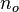$n_o$ n/a n/a
xy-plane xy-plane$n_o$ z$n_e$
xz-plane y$n_o$ xz-plane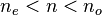$n_e < n < n_o$
other analogous to xz-plane

For a given propagation direction, in general there are two perpendicular polarizations for which the medium behaves as if it had a single effective refractive index.

In a uniaxial material, these polarizations are called the extraordinary and the ordinary ray (e and o rays), with the ordinary ray having the effective refractive index$n_o$.

A biaxial crystal is characterized by three refractive indices α, β, and γ applying to its principal axes.

A wave in a specified direction will consist of two polarization components with generally different effective refractive indices.

The so-called slow ray is the component for which the material has the higher effective refractive index (slower phase velocity), while the fast ray has a lower effective refractive index.Rays passing through a positively birefringent material. The incident light has parallel and perpendicular polarisation components (linear polarization at 45º the optic axis). The optic axis is perpendicular to the direction of the perpendicular component of incident ray, so the ray polarized parallel to the optic axis has a greater refractive index than the ray polarized perpendicular to it.

For a uniaxial material with the z axis defined to be the optical axis, the effective refractive indices are as in the table on the right. For rays propagating in directions other than z, the effective refractive index of the extraordinary ray is in between$n_o$ and$n_e$, depending on the angle between the polarization vector and the z axis. The effective refractive index can be determined using the index ellipsoid.

### Positive or negative

Uniaxial birefringence is classified as positive when the extraordinary index of refraction ne is greater than the ordinary index no. Negative birefringence means that Δn = ne – no is less than zero. In other words, the polarization of the fast (or slow) wave is perpendicular to the optical axis when the birefringence of the crystal is positive (or negative, respectively).

The terms “positive” and “negative” are not applied in the case of biaxial crystals, since all three of the principal axes have different refractive indices, rather than two being the same but different from the one that’s designated as the optic axis in a uniaxial crystal.

Biaxial materials, at 590 nm
Material Crystal system nα nβ nγ
borax Na2(B4O5)(OH)4·8(H2O) Monoclinic 1.447 1.469 1.472
epsom salt MgSO4·7(H2O) Monoclinic 1.433 1.455 1.461
mica, biotite K(Mg,Fe)
3
AlSi
3
O
10
(F,OH)
2
Monoclinic 1.595 1.640 1.640
mica, muscovite KAl2(AlSi3O10)(F,OH)2 Monoclinic 1.563 1.596 1.601
olivine (Mg, Fe)2SiO4 Orthorhombic 1.640 1.660 1.680
perovskite CaTiO3 Orthorhombic 2.300 2.340 2.380
topaz Al2SiO4(F,OH)2 Orthorhombic 1.618 1.620 1.627
ulexite NaCaB5O6(OH)6•5(H2O) Triclinic 1.490 1.510 1.520

### Biaxial birefringence

Biaxial birefringence, also known as trirefringence[citation needed], describes an anisotropic material in which the optical properties are not invariant under rotation about a particular axis (the optic axis, in uniaxial crystals). For such a material, the refractive index tensor n, will in general have three distinct eigenvalues that can be labeled nα, nβ and nγ.

## Measurement

Birefringence and other polarization based optical effects (such as optical rotation and linear or circular dichroism) can be measured by measuring the changes in the polarization of light passing through the material. These measurements are known as polarimetry.

Birefringence of lipid bilayers can be measured using dual polarisation interferometry. This provides a measure of the degree of order within these fluid layers and how this order is disrupted when the layer interacts with other biomolecules.

## ApplicationsReflective twisted nematic liquid crystal display. Light reflected by surface (6) (or coming from a backlight) is horizontally polarized (5) and passes through the liquid crystal modulator (3) sandwiched in between transparent layers (2,4) containing electrodes. Horizontally polarized light is blocked by the vertically oriented polarizer (1) except where its polarization has been rotated by the liquid crystal (3), appearing bright to the viewer

Birefringence is used in many optical devices.

Liquid crystal displays, the most common sort of flat panel display, cause their pixels to become lighter or darker through rotation of the polarization (circular birefringence) of linearly polarized light as viewed through a sheet polarizer at the screen’s surface.

Similarly, light modulators modulate the intensity of light through electrically induced birefringence of polarized light followed by a polarizer.

The Lyot filter is a specialized narrowband spectral filter employing the wavelength dependence of birefringence. Wave plates are thin birefringent sheets widely used in certain optical equipment for modifying the polarization state of light passing through it.

Birefringence also plays an important role in second harmonic generation and other nonlinear optical components, as the crystals used for this purpose are almost always birefringent. By adjusting the angle of incidence, the effective refractive index of the extraordinary ray can be tuned in order to achieve phase matching which is required for efficient operation of these devices.

### Medicine

Birefringence is utilized in medical diagnostics. One powerful accessory used with optical microscopes is a pair of crossed polarizing filters. Light from the source is polarized in the X direction after passing through the first polarizer, but above the specimen is a polarizer (a so-called analyzer) oriented in the Y direction. Therefore no light from the source will be accepted by the analyzer, and the field will appear dark. However areas of the sample possessing birefringence will generally couple some of the X polarized light into the Y polarization; these areas will then appear bright against the dark background. Modifications to this basic principle can differentiate between positive and negative birefringence.Urate crystals, with the crystals with their long axis seen as horizontal in this view being parallel to that of a red compensator filter. These appear as yellow, and are thereby of negative birefringence.

For instance, needle aspiration of fluid from a gouty joint will reveal negatively birefringent monosodium urate crystals. Calcium pyrophosphate crystals, in contrast, show weak positive birefringence. Urate crystals appear yellow and calcium pyrophosphate crystals appear blue when their long axes are aligned parallel to that of a red compensator filter, or a crystal of known birefringence is added to the sample for comparison.

Birefringence can be observed in amyloid plaques such as are found in the brains of Alzheimer’s patients when stained with a dye such as Congo Red.

Modified proteins such as immunoglobulin light chains abnormally accumulate between cells, forming fibrils. Multiple folds of these fibers line up and take on a beta-pleated sheet conformation. Congo red dye intercalates between the folds and, when observed under polarized light, causes birefringence.

In ophthalmology, scanning laser polarimetry utilises the birefringence of the optic nerve fibre layer to indirectly quantify its thickness, which is of use in the assessment and monitoring of glaucoma.

Birefringence characteristics in sperm heads allow for the selection of spermatozoa for intracytoplasmic sperm injection. Likewise, zona imaging uses birefringence on oocytes to select the ones with highest chances of successful pregnancy. Birefringence of particles biopsied from pulmonary nodules indicates silicosis.

### Stress induced birefringence

Isotropic solids do not exhibit birefringence. However, when they are under mechanical stress, birefringence results. The stress can be applied externally or is ‘frozen’ in after a birefringent plastic ware is cooled after it is manufactured using injection molding. When such a sample is placed between two crossed polarizers, colour patterns can be observed, because polarization of a light ray is rotated after passing through a birefingent material and the amount of rotation is dependent on wavelength. The experimental method called photoelasticity used for analyzing stress distribution in solids is based on the same principle.

### Other cases of birefringence

Birefringence is observed in anisotropic elastic materials. In these materials, the two polarizations split according to their effective refractive indices which are also sensitive to stress. The study of birefringence in shear waves traveling through the solid earth (the earth’s liquid core does not support shear waves) is widely used in seismology. Birefringence is widely used in mineralogy to identify rocks, minerals, and gemstones.

## Theory

Birefringence results when a material’s permittivity is not describable using a scalar value, but requires a tensor to relate the electric displacement (D) with the electric field (E).

Consider a plane wave propagating in an anisotropic medium, with a permittivity tensor ε and assuming no magnetic permeability in the medium:$\mu= \mu_0$. We shall assume that the electric field of a wave of angular frequency ω can be written in the form: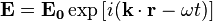$\mathbf{E=E_0}\exp \left[i(\mathbf{k \cdot r}-\omega t)\right] \,$ (2)

where r is the position vector, t is time, and E0 is a vector describing the electric field at r=0, t=0. Then we shall find the possible wave vectors k using Maxwell’s equations from which we obtain: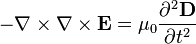$-\nabla \times \nabla \times \mathbf{E}= \mu_0 \frac{\part^2 \mathbf{D} }{\partial t^2}$ (3a)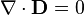$\nabla \cdot \mathbf{D} =0$ (3b)

where the so-called electric displacement vector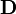$\mathbf D$ is now related to the electric field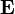$\mathbf E$ through the permittivity tensor ε:$\mathbf D = \epsilon \, \mathbf E \, .$

Substituting the definition of$\mathbf D$ and eqn. 2 into eqns. 3a-b leads to the conditions:$|\mathbf{k}|^2\mathbf{E_0}-\mathbf{(k \cdot E_0) k}= \mu_0 \omega^2 (\mathbf{\epsilon} \, \mathbf{E_0})$ (4a)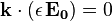$\mathbf{k} \cdot (\mathbf{\epsilon} \, \mathbf{E_0}) =0$ (4b)

Eqn. 4b indicates that$\mathbf D$ is orthogonal to the direction of the wavevector k, even though that is no longer generally true for$\mathbf E$ as would be the case in an isotropic medium.

To find the allowed values of k, E0 can be eliminated from eq 4a. If eqn 4a is written in Cartesian coordinates with the x, y and z axes chosen in the principal directions of the permitivity tensor ε, then$\mathbf{\epsilon}= \epsilon_0 \begin{bmatrix} n_x^2 & 0 & 0 \\ 0& n_y^2 & 0 \\ 0& 0& n_z^2 \end{bmatrix} \,$ (4c)

where the diagonal values are squares of the refractive indices for polarizations along the three principal axes x, y and z. With ε in this form, and noting that the speed of light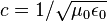$c = 1/ \sqrt{\mu_0 \epsilon_0}$, eqn. 4a becomes$\left(-k_y^2-k_z^2+\frac{\omega^2n_x^2}{c^2}\right)E_x + k_xk_yE_y + k_xk_zE_z =0$ (5a)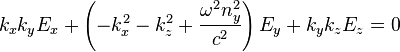$k_xk_yE_x + \left(-k_x^2-k_z^2+\frac{\omega^2n_y^2}{c^2}\right)E_y + k_yk_zE_z =0$ (5b)$k_xk_zE_x + k_yk_zE_y + \left(-k_x^2-k_y^2+\frac{\omega^2n_z^2}{c^2}\right)E_z =0$ (5c)

where Ex, Ey, Ez, kx, ky and kz are the components of E0 and k. This is a set of linear equations in Ex, Ey, Ez, and they have a non-trivial solution if the following determinant is zero: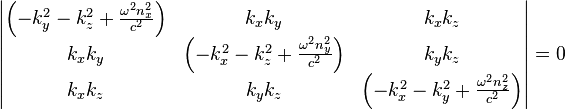$\begin{vmatrix} \left(-k_y^2-k_z^2+\frac{\omega^2n_x^2}{c^2}\right) & k_xk_y & k_xk_z \\ k_xk_y & \left(-k_x^2-k_z^2+\frac{\omega^2n_y^2}{c^2}\right) & k_yk_z \\ k_xk_z & k_yk_z & \left(-k_x^2-k_y^2+\frac{\omega^2n_z^2}{c^2}\right) \end{vmatrix} =0\,$ (6)

Evaluating the determinant of eqn (6), and rearranging the terms, we obtain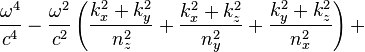$\frac{\omega^4}{c^4} - \frac{\omega^2}{c^2}\left(\frac{k_x^2+k_y^2}{n_z^2}+\frac{k_x^2+k_z^2}{n_y^2}+\frac{k_y^2+k_z^2}{n_x^2}\right) +$$\left(\frac{k_x^2}{n_y^2n_z^2}+\frac{k_y^2}{n_x^2n_z^2}+\frac{k_z^2}{n_x^2n_y^2}\right)(k_x^2+k_y^2+k_z^2)=0$

In the case of a uniaxial material, choosing the optic axis to be in the z direction so that nx=ny=no and nz=ne, this expression can be factored into$\left(\frac{k_x^2}{n_o^2}+\frac{k_y^2}{n_o^2}+\frac{k_z^2}{n_o^2} -\frac{\omega^2}{c^2}\right)\left(\frac{k_x^2}{n_e^2}+\frac{k_y^2}{n_e^2}+\frac{k_z^2}{n_o^2} -\frac{\omega^2}{c^2}\right)=0\,.$ (8)

Setting either of the factors in eqn 8 to zero will define an ellipsoidal surface in space of allowed wave vectors k. The first factor being zero defines a sphere corresponding to ordinary rays, in which the effective refractive index is exactly no. The second defines a spheroid symmetric about the z axis. This solution corresponds to extraordinary rays in which the effective refractive index is in between no and ne. Therefore for any arbitrary direction of propagation, two distinct wavevectors k are allowed corresponding to the polarizations of the ordinary and extraordinary rays. A general state of polarization launched into the medium can be decomposed into two such waves which will then propagate with different k vectors (except in the case of propagation in the direction of the optic axis). For a biaxial material a similar but somewhat more complicated condition on the two waves can be described.